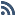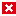# Time Conversion problemGuest

Search

— Forum Scope —

— Match —

— Forum Options —

Minimum search word length is 3 characters - maximum search word length is 84 charactersTime Conversion problemraihan84
Member
Forum Posts: 12
Member Since:
March 4, 2011Offline

Hello guys,

I have got a problem. I wanna change an integer number to corresponding hours and minutes. For example, if you enter 100 then it will return 1:40 hours, that is one hour and 40 minutes.

I like to have and user defined functions or macro code..............

Thanks
RaihanMod
Forum Posts: 867
Member Since:
August 11, 2011Offline

So...I'm not a macro coder, but your logic would look like this:

x = input number

if x < 60 return "x minutes"
else
y = x / 60 --gives number of hours
z = x-y -- gives number of minutes
return "y hours and z minutes"raihan84
Member
Forum Posts: 12
Member Since:
March 4, 2011Offline

So...I'm not a macro coder, but your logic would look like this:

x = input number

if x < 60 return "x minutes"
else
y = x / 60 --gives number of hours
z = x-y -- gives number of minutes
return "y hours and z minutes"[/quote:1bahq5ps]

Yeh, you have got my logic but I wanna return as y:z format, where y is hours and z is min.raihan84
Member
Forum Posts: 12
Member Since:
March 4, 2011Offline

I have got two ways to solve this problem.................................

Let A1 = 100, now if we want to return value at A2 cell then at formula bar I have written the code

1. [code:3h4zvu1y]CONCATENATE(FLOOR(A1/60,1), ":",(A1/60-FLOOR(A1/60,1))*60)[/code:3h4zvu1y]
2. [code:3h4zvu1y]INT(A1/60)&":"&MOD(A1,60)[/code:3h4zvu1y]

in return in cell A2 i got 1:40.

Does anybody know any other formulas?Mod
Forum Posts: 867
Member Since:
August 11, 2011Offline

This will handle values under 60:
[code:29xp43hs]=IF(A1>60,CONCATENATE(TRUNC(A1/60), ":", A1-(TRUNC(A1/60)*60)),CONCATENATE("0:", A1))[/code:29xp43hs]raihan84
Member
Forum Posts: 12
Member Since:
March 4, 2011Offline

This will handle values under 60:
[code:1rdot3s4]=IF(A1>60,CONCATENATE(TRUNC(A1/60), ":", A1-(TRUNC(A1/60)*60)),CONCATENATE("0:", A1))[/code:1rdot3s4][/quote:1rdot3s4]

Thanks Zigraihan84
Member
Forum Posts: 12
Member Since:
March 4, 2011Offline

This could be another formula.....................

[code:1hx7264w]TEXT(FLOOR(A1/60,1),"00")&":"&TEXT(MOD(A1,60),"00")[/code:1hx7264w]

ThanksCarol Bratt
Mod
Forum Posts: 66
Member Since:
August 11, 2011Offline

If you have a time in a decimal format, i.e. an integer and a fraction, e.g. 2.5 hours (2 and a half hours) and you want to convert to a standard time format 02:30 (2 hours and 30 minutes), then you can use a formula based on the TIME function with the following syntax:
TIME(hours,minutes,seconds)
Example:
We have a decimal time 2.5 in cell A2. we can use the following formula to convert it to a standard time:
=TIME(INT(A2);(A2-INT(A2))*60;0)raihan84
Member
Forum Posts: 12
Member Since:
March 4, 2011Offline

If you have a time in a decimal format, i.e. an integer and a fraction, e.g. 2.5 hours (2 and a half hours) and you want to convert to a standard time format 02:30 (2 hours and 30 minutes), then you can use a formula based on the TIME function with the following syntax:
TIME(hours,minutes,seconds)
Example:
We have a decimal time 2.5 in cell A2. we can use the following formula to convert it to a standard time:
=TIME(INT(A2);(A2-INT(A2))*60;0)Carol Bratt
Mod
Forum Posts: 66
Member Since:
August 11, 2011Offline
Forum Timezone: America/Indiana/Indianapolis

Most Users Ever Online: 188

Currently Online:
19 Guest(s)

1 Guest(s)

Member Stats:

Guest Posters: 10

Members: 2075

Moderators: 6

Forum Stats:

Groups: 8

Forums: 19

Topics: 1755

Posts: 12768

Administrators: Jim Hillier, Richard Pedersen, David Hartsock, Marc Thomas

Moderators: Judy Novotny, dandl, Jason Shuffield, Jim Canfield, Dick Evans, Sergey Grankin